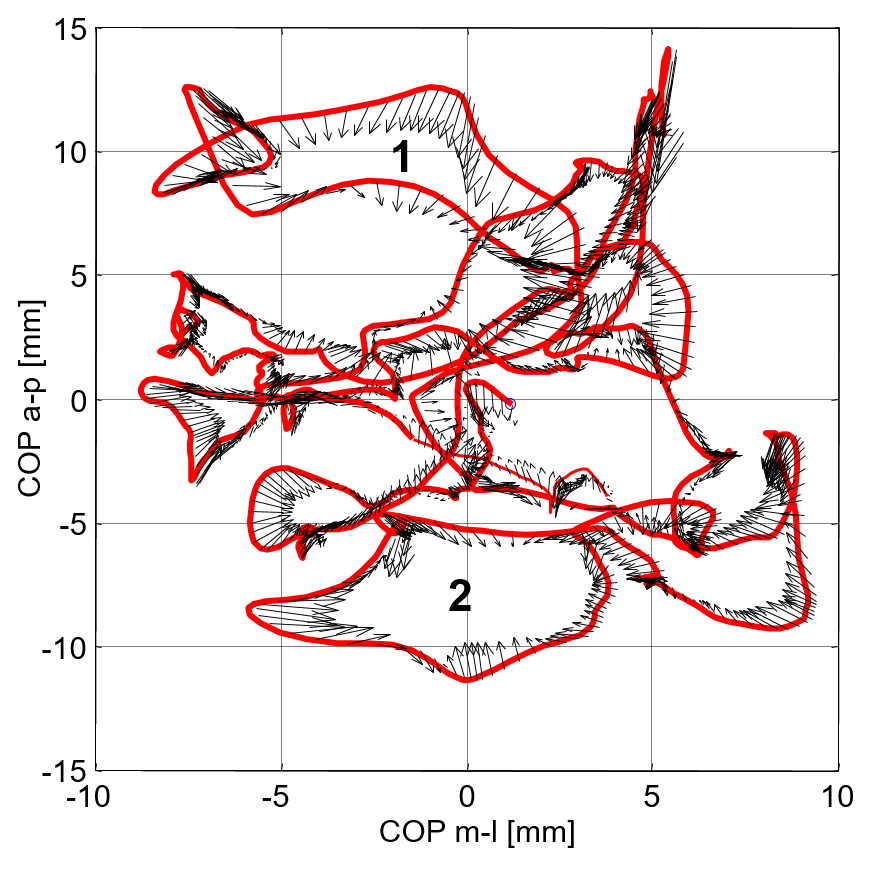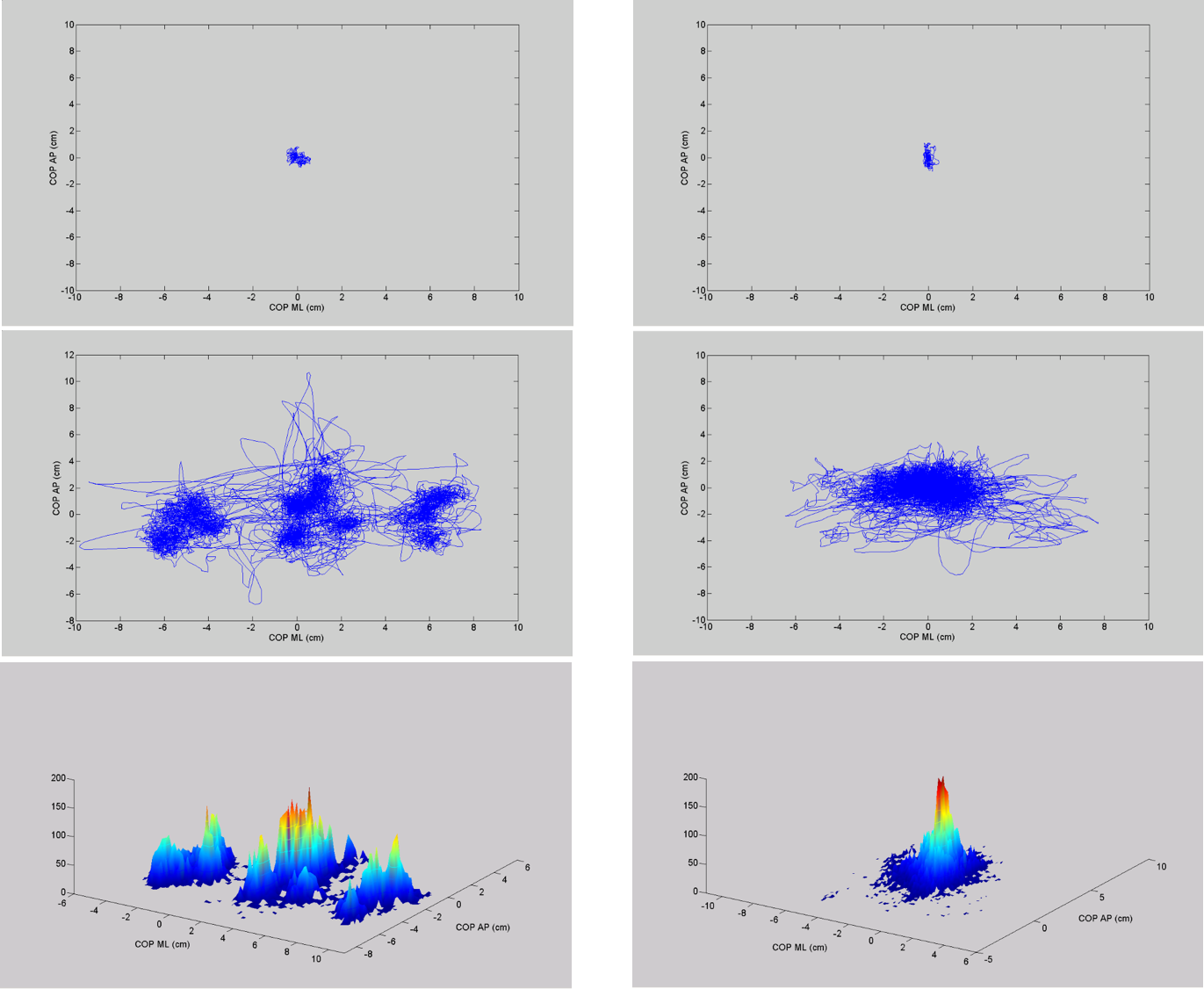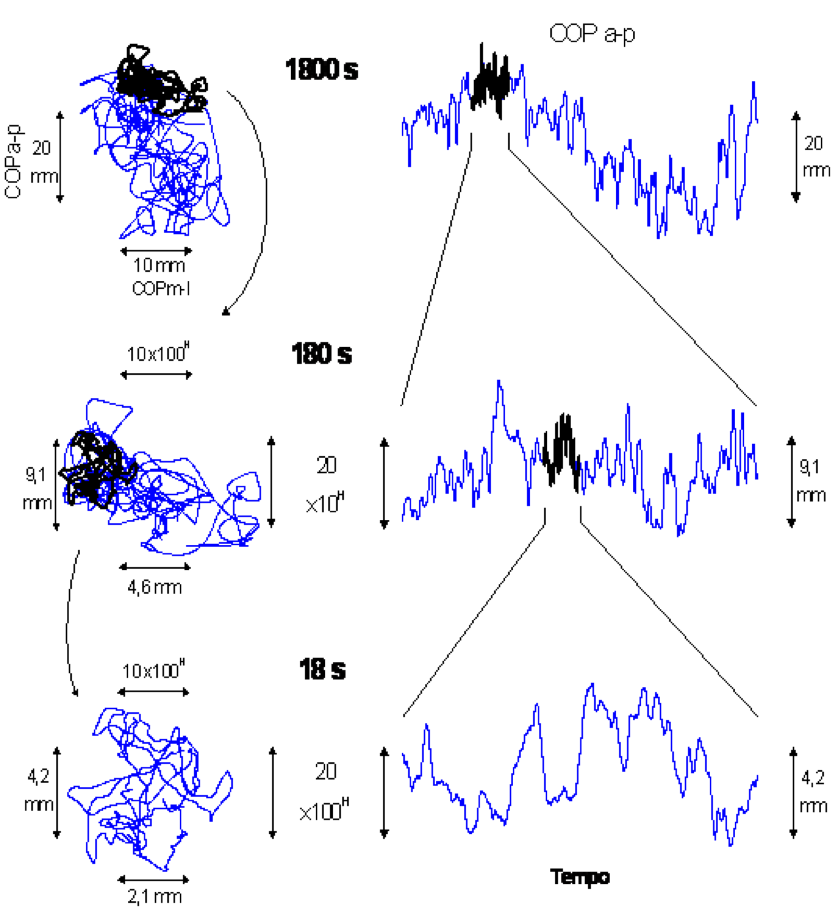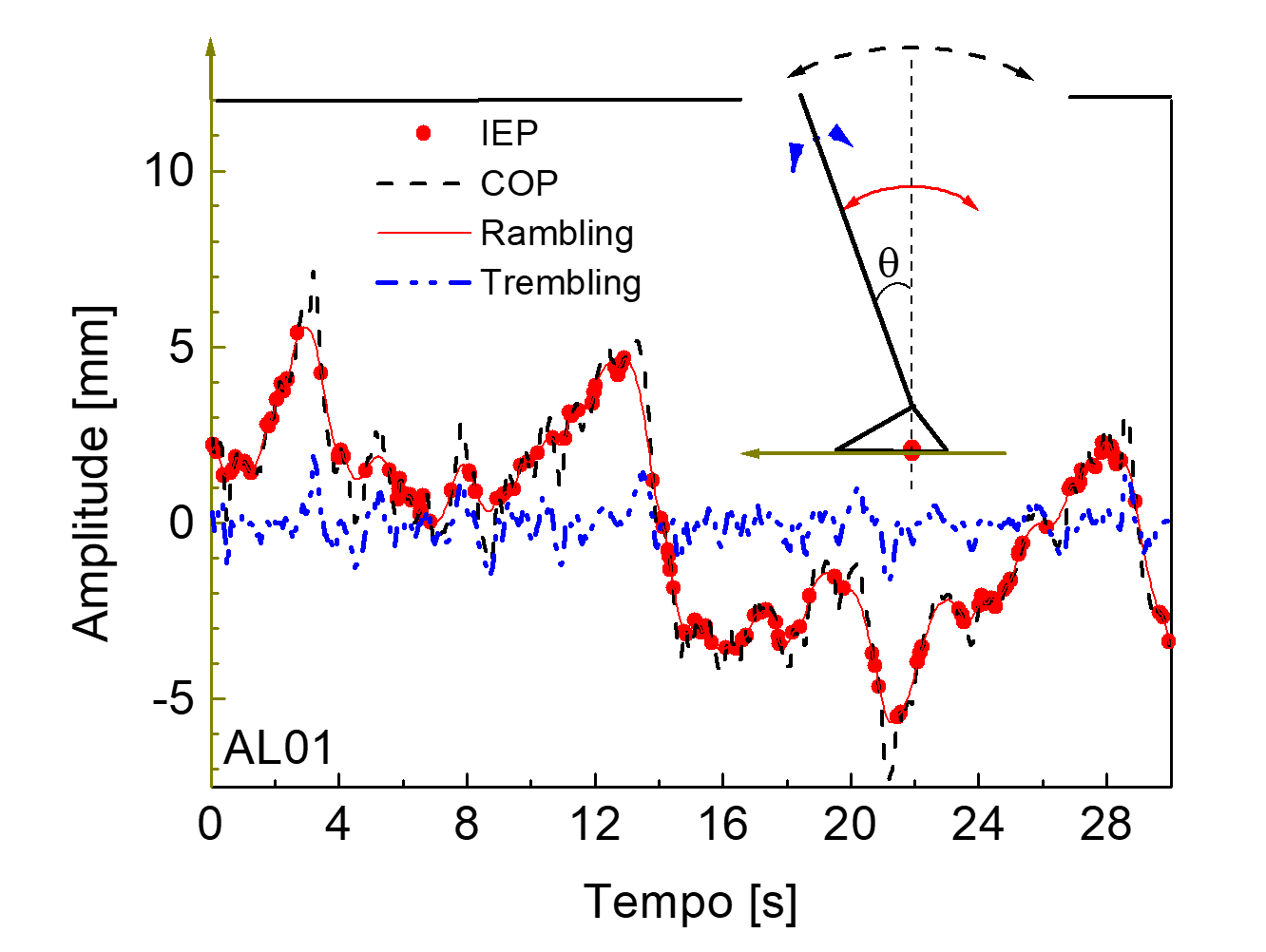# Postural Sway: Rambling and Trembling decomposition of the COP¶

Marcos Duarte
Laboratory of Biomechanics and Motor Control (http://demotu.org/)
Federal University of ABC, Brazil

### First, let's import the necessary Python libraries and configure the environment¶

In :
import sys, os
sys.path.insert(1, r'./../functions')
import numpy as np
import pandas as pd
pd.set_option('precision', 4)
# matplotlib configuration:
import matplotlib
import matplotlib.pyplot as plt
import matplotlib.gridspec as gridspec
%matplotlib inline
import seaborn as sns
sns.set_context("notebook", font_scale=1.4,
rc={'font.size': 16, 'lines.linewidth': 2,\
'lines.markersize': 10, 'axes.titlesize': 'x-large'})
matplotlib.rc('legend', numpoints=1, fontsize=16)
# IPython:
from IPython.display import display, Image, IFrame
import ipywidgets
from ipywidgets import FloatProgress, interactive


### Hypothesis about the human postural control (Zatsiorsky)¶

The control system for equilibrium of the human body includes two subsystems:

1. the first one determining a reference position with respect to which the body equilibrium is maintained.
2. the second one maintaining equilibrium about the pre-selected reference point.

### Findings supporting a two-subsystems control¶

Gurfinkel et al. (1995) Kinesthetic reference for human orthograde posture. Neuroscience, 68, 229.

The supporting surface where the subjects stood was tilted slowly (0.04 $^o/s$).
During the tilting, small high frequency oscillations of the body were superimposed on a large slow body movements.
The usual process of stabilization of the body continued, but the instant equilibrium was maintained relative to a slowly changing position, rather than around a fixed set point.

See Zatsiorsky & Duarte (2000), for a review about findings supporting a two-subsystems control.

### Force fields during quiet standing¶

In :
Image(filename='../images/forcevectors.png', width=500)

Out:### Prolonged Standing¶

In :
display(Image(filename='../images/prolongedstanding.png', width=600))### Postural sway: a fractal process¶

In :
display(Image(filename='../images/fractal.png', width=500))
print('Duarte & Zatsiorsky (2000)')Duarte & Zatsiorsky (2000)


### Rambling and Trembling decomposition of the COP¶

The reference point migration is called rambling and the COP migration around the reference was coined trembling.

• Zatsiorsky VM, Duarte M (1999) Instant equilibrium point and its migration in standing tasks: Rambling and trembling components of the stabilogram. Motor Control, 3, 28. PDF.
• Zatsiorsky VM, Duarte M (2000) Rambling and trembling in quiet standing. Motor Control, 2, 185. PDF.
In :
IFrame('http://demotu.org/pubs/mc99b.pdf', width='100%', height=450)

Out:
In :
IFrame('http://demotu.org/pubs/mc00.pdf', width='100%', height=450)

Out:

### Rambling and Trembling during quiet standing¶

In :
Image(filename='../images/rambtremb.png', width=600)

Out:### Balance maintenance according to the Rambling and Trembling hypothesis¶

1. The CNS specifies an intended position of the body. The intended position is specified by a reference point on the supporting surface with respect to which body equilibrium is instantly maintained.
2. The reference point migrates and can be considered a moving attracting point.
3. The body sways because of two reasons: the migration of the reference point and the deviation away from the reference point.
4. When the deflection is not too large, the restoring force is due to the ‘apparent intrinsic stiffness’ of the muscles.

### Decomposition based on the instant-equilibrium-point hypothesis¶

The rambling and trembling components of the COP trajectory were computed in the following way:

1. The instants when $F_{hor}$ changes its sign from positive (negative) to negative (positive) are determined.
2. The COP positions at these instants (the instant equilibrium points, IEP, or zero-force points) are determined.
3. To obtain an estimate of the rambling trajectory, the IEP discrete positions are interpolated by cubic spline functions.
4. To obtain the trembling trajectory, the deviation of the COP from the rambling trajectory is determined.

### Some auxiliary functions¶

In :
def detect_zerocross(y, method='no_zero'):
"""
Zero-crossing detection, index before a zero cross.
"""

import numpy as np

y = np.asarray(y)

if method == 'no_zero':
# doesn't detect if a value is exactly zero:
inds0 = np.where(y[:-1] * y[1:] < 0)
else:
# detects if a value is exactly zero:
inds0 = np.where(np.diff(np.signbit(y)))

return inds0

In :
def iep_decomp(time, force, cop):
"""
Center of pressure decomposition based on the IEP hypothesis.
"""

import numpy as np
from scipy.interpolate import UnivariateSpline

force = force - np.mean(force)

# detect zeros
inds0 = detect_zerocross(force)

# select data between first and last zeros
time = time[inds0:inds0[-1]+1]
force = force[inds0:inds0[-1]+1]
cop = cop[inds0:inds0[-1]+1]

# IEP0 & IEP:
iep0 = inds0 - inds0
spl = UnivariateSpline(time[iep0], cop[iep0], k=3, s=0)
rambling = spl(time)
trembling = cop - rambling

return rambling, trembling, iep0, inds0


### Load some postural sway data¶

In :
# data available at https://doi.org/10.6084/m9.figshare.3394432.v2
# locally:
path2 = r'./../../../X/BDB/'
filename = os.path.join(path2, 'BDS00038.txt')
names=['Time','Fx','Fy','Fz','Mx','My','Mz','COPx','COPy'],
engine='c')

t, Fx, Fy, Fz, Mx, My, Mz, COPx, COPy = [_ for _ in grf.values.T]

In :
def plot_grf(grf):
t, Fx, Fy, Fz, Mx, My, Mz, COPx, COPy = [_ for _ in grf.values.T]
Funits, Munits, COPunits = 'N', 'Nm', 'cm'
plt.figure(figsize=(12, 7))

gs1 = gridspec.GridSpec(3, 1)
gs1.update(bottom=0.52, top=0.96, hspace=0.12, wspace=.2)
ax1, ax2, ax3 = plt.subplot(gs1), plt.subplot(gs1), plt.subplot(gs1)
gs2 = gridspec.GridSpec(3, 3)
gs2.update(bottom=0.08, top=0.42, wspace=0.3)
ax4, ax5 = plt.subplot(gs2[:, :-1]), plt.subplot(gs2[:, 2])

ax1.set_ylabel('Fx (%s)' %Funits)
ax1.set_xticklabels([]), ax1.locator_params(axis='y', nbins=4)
ax1.yaxis.set_label_coords(-.07, 0.5)
ax2.set_ylabel('Fy (%s)' %Funits)
ax2.set_xticklabels([]), ax2.locator_params(axis='y', nbins=4)
ax2.yaxis.set_label_coords(-.07, 0.5)
ax3.set_ylabel('Fz (%s)' %Funits)
ax3.locator_params(axis='y', nbins=4)
ax3.yaxis.set_label_coords(-.07, 0.5)
ax3.set_xlabel('Time (s)')
ax4.set_xlabel('Time (s)')
ax4.set_ylabel('COP (%s)' %COPunits)
ax5.set_xlabel('COPml (%s)' %COPunits)
ax5.set_ylabel('COPap (%s)' %COPunits)

ax1.plot(t, Fx), ax2.plot(t, Fy), ax3.plot(t, Fz)
ax4.plot(t, COPx, 'b', label='COP ap')
ax4.plot(t, COPy, 'r', label='COP ml')
ax4.yaxis.set_label_coords(-.1, 0.5)
ax4.legend(fontsize=12, loc='best', framealpha=.5)
ax5.plot(COPy, COPx)
ax5.locator_params(axis='both', nbins=5)
plt.suptitle('Ground reaction force data during quiet standing', fontsize=20, y=1)
plt.show()

In :
plot_grf(grf)